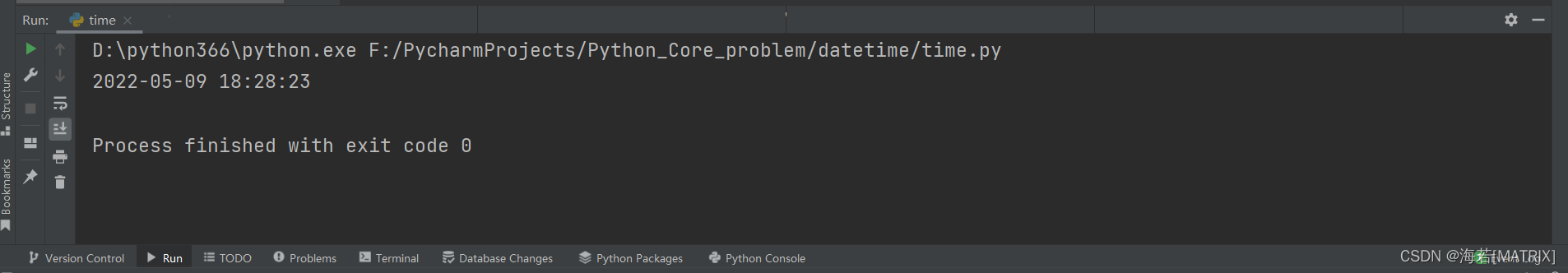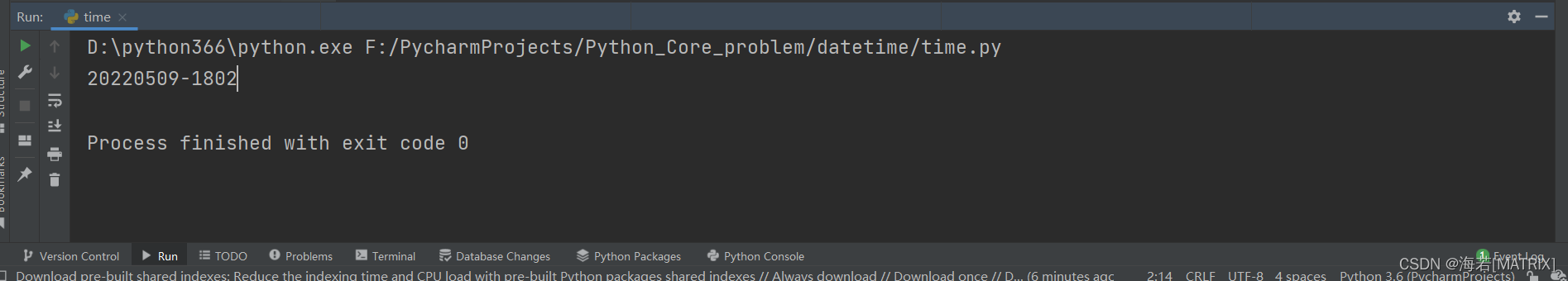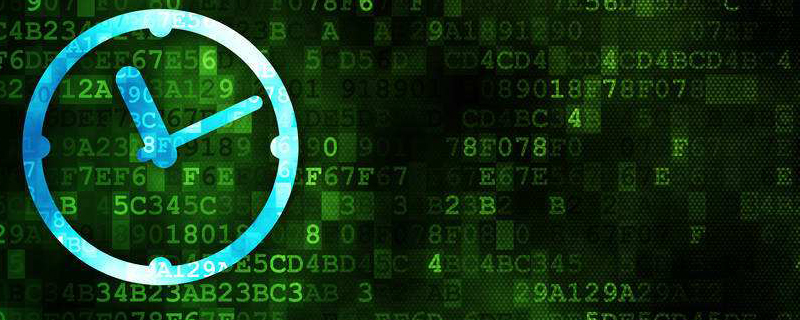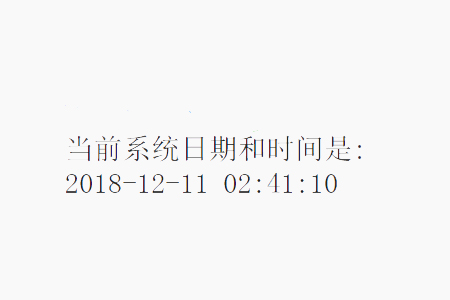• python获取当前系统时间
千次阅读
2019-07-31 19:09:00

# python获取当前系统时间

import datetime
time = datetime.datetime.now().strftime('%Y-%m-%d %H:%M:%S')
print(time)

# 结果
# 2019-07-31 19:08:17

转载于:https://www.cnblogs.com/17vv/p/11278480.html

更多相关内容
• start = datetime.now() #获取当前系统时间 获取当前系统时间的输出结果 *Out:*datetime.datetime(2021, 11, 16, 11, 33, 12, 3000) 方法2： import time start = time.localtime(time.time())))##获取当前系统...

方法1：

from datetime import datetime
start = datetime.now()  #获取当前系统时间


获取当前系统时间的输出结果
*Out:*datetime.datetime(2021, 11, 16, 11, 33, 12, 3000)
方法2：

import time
start = time.localtime(time.time())))##获取当前系统时间



Out: time.struct_time(tm_year=2021, tm_mon=11, tm_mday=16, tm_hour=11, tm_min=37, tm_sec=47, tm_wday=1, tm_yday=320, tm_isdst=0)

strftime( )函数
Python内置的strftime( )函数：实现本地时间\日期的格式化（将任意格式的日期字符串按要求进行格式化）

start_time1 =  start.strftime('%Y-%m-%d %H:%M:%S')
start_time2 =  start.strftime('%m,%d,%Y') ##中间的连接符可以为“-”也可以为“/”，也可以为其他符号
print(start_time1,start_time2)

##输出结果('2021-11-16 11:36:42', '11,16,2021')


时间暂停一秒

import time

print (time.strftime('%Y-%m-%d %H:%M:%S',time.localtime(time.time())))

# 暂停一秒
time.sleep(1)

print (time.strftime('%Y-%m-%d %H:%M:%S',time.localtime(time.time())))

展开全文python
• 1.python获取当前时间代码 import datetime current_time = datetime.datetime.now() print("current_time: " + str(current_time)) 2.执行结果 current_time: 2021-08-11 11:48:11.122521## 1.python获取当前时间代码

import datetime

current_time = datetime.datetime.now()
print("current_time:    " + str(current_time))


## 2.执行结果


current_time:    2021-08-11 11:48:11.122521



## 3.格式化输出系统时间-1

import time

print(time.strftime('%Y-%m-%d %H:%M:%S', time.localtime(time.time())))## 4.格式化输出系统时间-2

timestr = time.strftime("%Y%m%d-%H%M%S")
print (timestr)## 5.格式化符号

%x本地相应的日期表示%X本地相应的时间表示
%z当前时区的名称
%%号本身

%y两位数的年份表示（00-99）
%Y四位数的年份表示（000-9999）

%m月份（01-12）
%d月内中的一天（0-31）

%H24小时制小时数（0-23）
%I 12小时制小时数（01-12）

%M分钟数（00=59）
%S秒（00-59）

%a本地简化星期名称%A本地完整星期名称
%b本地简化的月份名称%B本地完整的月份名称
%c本地相应的日期表示和时间表示%j年内的一天（001-366）
%p本地A.M.或P.M.的等价符su一年中的星期数（00-53）星期天为星期的开始
%w星期（0-6），星期天为星期的开始W一年中的星期数（00-53）星期一为星期的开始


展开全文python 人工智能
• python获取当前系统时间，包括年月日，时分秒，主要通过Python中的datetime模块来实现。下面我们就通过具体的代码示例，给大家详细介绍Python获取当前时间日期的实现方法。代码示例如下：import datetimenow = ...

python获取当前系统时间，包括年月日，时分秒，主要通过Python中的datetime模块来实现。下面我们就通过具体的代码示例，给大家详细介绍Python获取当前时间日期的实现方法。

代码示例如下：import datetime

now = datetime.datetime.now()

print ("当前系统日期和时间是: ")

print (now.strftime("%Y-%m-%d %H:%M:%S"))

获取当前系统时间，结果如下所示：Python datetime:

datetime模块提供了以简单和复杂的方式操作日期和时间的类。

datetime包括类如下：

date-日期对象,常用的属性有year, month, day

time-时间对象

datetime-日期时间对象,常用的属性有hour, minute, second, microsecond

datetime_CAPI-日期时间对象C语言接口

timedelta-时间间隔，即两个时间点之间的长度

tzinfo-时区信息对象。

now(tz=None)返回当前的本地日期和时间。如果可选参数tz没有指定，与today()一样。

strftime(format)返回一个表示日期的字符串，由显式格式字符串控制。引用小时、分钟或秒的格式代码将看到0值。

本篇文章就是关于Python获取当前系统时间及日期的方法介绍，也很简单易懂，希望对需要的朋友有所帮助！

展开全文• 首先import time time.strftime('%Y-%m-%d %H:%M:%S', time.localtime(time.time()))python
• python 如何获取当前系统时间 <script> \$(".postTitle2").removeClass("postTitle2").addClass("singleposttitle"); </script> </div> 1.导入包　import datetime 2.获取当前的时间 curr_...
• python获取当前系统时间，主要通过Python中的datetime模块来实现。 import datetime from time import strftime 获取当前时间 now=datetime.datetime.now() print(now.strftime("%Y-%m-%d %H:%M:%S")) 获取当前...python
• from time import time # 引入time模块 now = time() print "当前时间戳为:", now 参考： Python 日期和时间：https://www.runoob.com/python/python-date-time.htmlpytorch 人工智能
• 我有的时候写程序要用到当前时间，我就想用python去取当前时间，虽然不是很难，但是老是忘记，用一次丢一次，为了能够更好的记住，我今天特意写下python 当前时间这篇文章，如果你觉的对你有用的话，可以收藏下。...
• 取得当前时间戳import timeprint time.time()格式化时间戳为标准格式print time.strftime('%Y.%m.%d',time.localtime(time.time()))获取30天前的时间(通过加减秒数来获取现在或者未来某个时间点)print time.strftime...
• 来源：http://www.oldboyedu.com/zuixin_wenzhang/index/id/131.html在从事Python相关工作中，... time模块**time模块下有很多函数可以日期和时间的处理，如time.time()用于获取当前时间戳，localtime()将浮点数的时...
• time.strftime('%Y-%m-%d',time.localtime(time.time()))python
• 如何在python获取以毫秒为单位的当前时间？import time; ms = time.time()*1000.0@samplebias:time.time()在某些平台和python版本上的精度可能比datetime.utcnow()差。从什么时候开始以毫秒为单位？如果您的意思是...
• python获取系统当前时间的方法：可以利用datetime.datetime.now()函数来获取。具体方法：1、导入datetime包；2、获取当前时间，如【curr_time = datetime.datetime.now()】。具体方法：1、导入包import datetime...
• datetime：日期时间模块，提供多种方法操作日期和时间 import datetime today=datetime.date.today() print today 输出为： 2020-05-01python
• ## Python获取系统时间

万次阅读 2017-04-17 22:59:01
Python获取系统时间  Python获取系统时间方法： 1. 导入模块： import datetime 2. 获取当前时间： theTime = datetime.datetime.now() 得到的时间格式是： 2017-04-17 22:46:18.013458  ...python python获取时间
• import time import datetime t1=time.time() print(t1) time.sleep(0.2) t2=time.time() print(t2) print("相差",(datetime.datetime.fromtimestamp(t2)-datetime.datetime.fromtimestamp(t1)).microseconds/1000....python
• 导入库：import datetime获取当前日期和时间：now_time = datetime.datetime.now()格式化成我们想要的日期：strftime()比如：“2016-09-21”：datetime.datetime.now().strftime('%Y-%m-%d')在当前时间增加1小时：...
• import time print('时间显示') for i in range(3): print(time.strftime('%Y-%m-%d %H:%M:%S',time.localtime(time.time()))) time.sleep(1) #每隔1秒打印一次系统时间，打印三次 #time.time() 返回当前时间的...
• import datetime def get_datetime_str(style='dt'): cur_time = datetime.datetime.now() date_str = cur_time.strftime('%y%m%d') time_str = cur_time.strftime('%H%M%S') if style == 'data': ...python
• 秒级 import time now = time.time() #返回float数据 # 获取当前时间戳---秒级级 print(int(now)) 毫秒级 import time now = time.time() #返回float数据 #毫秒级时间戳 print(int(round(now * 1000)))python
• 因为这天呀发生了一件大事，UNIX操作系统诞生了，这UNIX诞生可有来历，他是一个歪果仁利用老婆孩子外出度假的时间来完成的，我假设大家了解操作系统的复杂性，那么我们预估他老婆要外出几年才能完成UNIX系统的编写？...
• 获取当前日期和时间：now_time = datetime.datetime.now()3.格式化成我们想要的日期：strftime()比如：“2016-09-21”：datetime.datetime.now().strftime('%Y-%m-%d')4.在当前时间增加1小时：add_hour=datetime....
• ## python获取当前时间

万次阅读 2019-04-23 11:08:54
Python 程序能用很多方式处理日期和时间，转换日期格式是一个常见的功能。 每个时间戳都以自从1970年1月1日午夜（历元）经过了多长时间来表示。 当前时间-时间戳 #!/usr/bin/python # -*- coding: UTF-8 -*-...python...